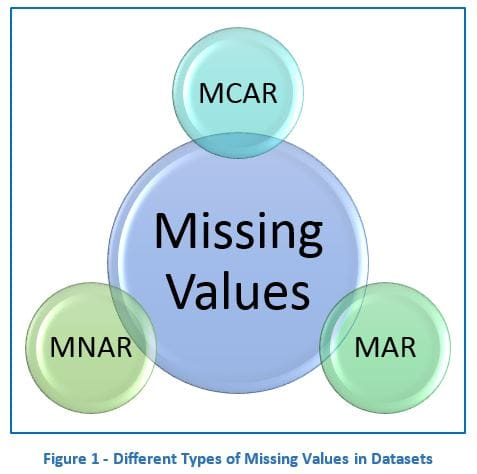# Appropriately Handling Missing Values for Statistical Modelling and Prediction

Many statisticians in industry agree that blindly imputing the missing values in your dataset is a dangerous move and should be avoided without first understanding why the data is missing in the first place.Missing values are an eventual reality in almost every dataset. Sometimes it may even be a cause for concern if there were no missing values just because you expect there to be data missing. Dealing with missing values have been a subject of debate for decades among statisticians due to it compromising the reliability of sample studies if left unchecked or incorrectly dealt with. In many university courses, students are simply taught to impute the missing values with the mean for continuous data, median for categorical data or to simply remove rows with missing values if it is just a small representation of your dataset (heuristically, less than 10% has been the threshold). Many statisticians in industry agree that blindly imputing the missing values in your dataset is a dangerous move and should be avoided without first understanding why the data is missing in the first place.

In 1976, a paper by Donald B. Rubin (Inference and Missing Data) suggested there are 3 assumptions for the presence of missing values in your dataset are:

• Missing Completely At Random (MCAR)
• Missing completely at random (MCAR) is defined as when the probability that the data are missing is not related to either the specific value which is supposed to be obtained or the set of observed responses. However, if data are missing by design, because of an equipment failure or because the samples are lost in transit or technically unsatisfactory, such data are regarded as being MCAR. The statistical advantage of data that are MCAR is that the analysis remains unbiased. Power may be lost in the design, but the estimated parameters are not biased by the absence of the data.
• Example: If we take the relationship between age and income and there was income data missing, the missing data is MCAR is there is no relationship between the missing income data and the age of the participants. There is no pattern to the missing data.
• Missing At Random (MAR)
• Data are regarded to be MAR when the probability that the responses are missing depends on the set of observed responses, but is not related to the specific missing values which is expected to be obtained. As we tend to consider randomness as not producing bias, we may think that MAR does not present a problem. However, MAR does not mean that the missing data can be ignored. If a dropout variable is MAR, we may expect that the probability of a dropout of the variable in each case is conditionally independent of the variable, which is obtained currently and expected to be obtained in the future, given the history of the obtained variable prior to that case.
• Missing Not At Random (MNAR)
• If the characters of the data do not meet those of MCAR or MAR, then they fall into the category of missing not at random (MNAR). The cases of MNAR data are problematic. The only way to obtain an unbiased estimate of the parameters in such a case is to model the missing data. The model may then be incorporated into a more complex one for estimating the missing values.

### How to Handle Missing Values

• Listwise Deletion (Complete Case Analysis)
• This is the most simple and less time consuming method of all. It simply means to just conduct your analysis on rows of data that have no missing values. This is sadly default behaviour in most academic settings when your student learning data preprocessing. This should only be used if we can confirm our missing data is MCAR. If not our analysis results cannot be unbiased. In reality, this is more significant in industries like pharma than other industries where it is common practice. If there is a large enough sample, where power is not an issue, and the assumption of MCAR is satisfied, the listwise deletion may be a reasonable strategy. However, when there is not a large sample, or the assumption of MCAR is not satisfied, the listwise deletion is not the optimal strategy.
• Pairwise Deletion (Available Case Analysis)
• In this scenario, instead of removing data like in Listwise Deletion, Pairwise Deletion calls for conducting analysis using the rows and columns that do not have any missing values and omitting the ones that do. This healps to retain data and improve statistical power, however now analysis cannot be compared to due to varying sample sizes and biased standard errors. Pairwise deletion is known to be less biased for the MCAR or MAR data. However, if there are many missing observations, the analysis will be deficient.
• Statistical Imputation
• Imputation involves replacing missing values with substituted values obtained from a statistical analysis to produce a complete data set without missing values for analysis. Imputations can be created by using either an explicit or an implicit modeling approach. The explicit modeling approach assumes that variables have a certain predictive distribution and estimates the parameters of each distribution, which is used for imputations. It includes different methods of imputation by mean, median, probability, ratio, regression, predictive-regression, and assumption of distribution.
• In these cases, using a point estimate such as mean and median are commonly used but are highly unreliable mostly due to data skewness and this method is more suited towards data that resemble a normal distribution (which is unlikely on real world data). Real world data is often skewed which make descriptive statistics like mean and median less useful

Related

By subscribing you accept KDnuggets Privacy Policy

By subscribing you accept KDnuggets Privacy Policy

By subscribing you accept KDnuggets Privacy Policy